## 2008年10月31日 星期五

### Oct.26, 公館小吃 藍家割包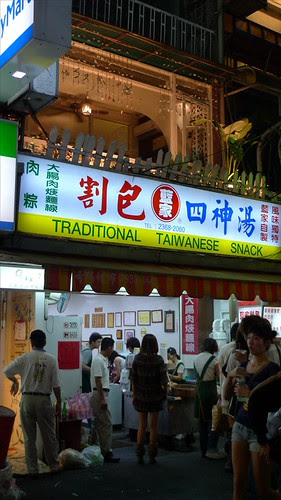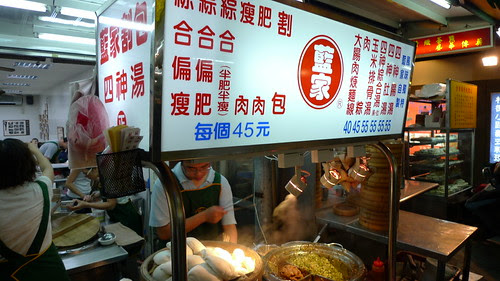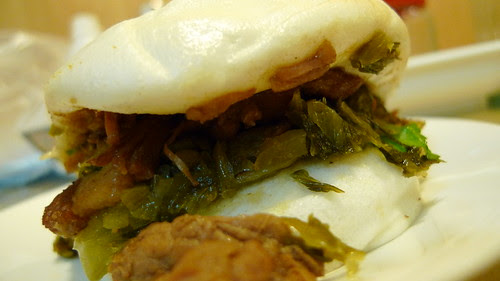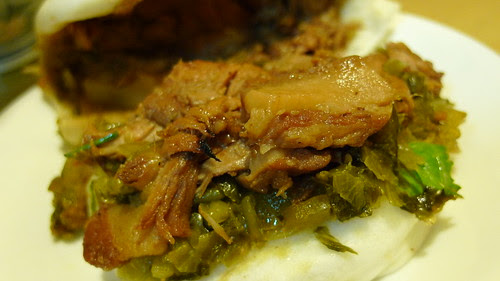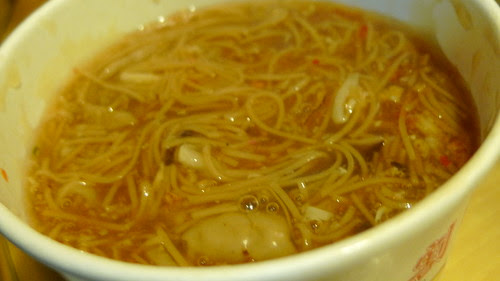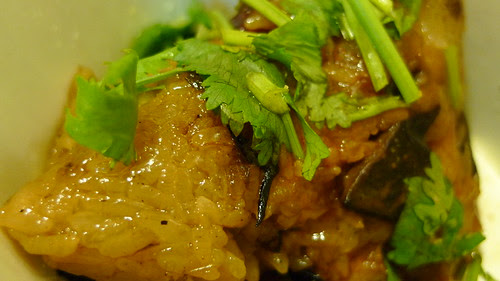(02)2368-2060

## 2008年10月26日 星期日

### [轉貼]有趣的計算

Hard work（努力工作）
H+A+R+D+W+O+R+K=
8+1+18+4+23+15+18+11=98%

Knowledge（知識）
K+N+O+W+L+E+D+G+E=
11+14+15+23+12+5+4+7+5=96%

Love（愛情）
L+O+V+E=12+5+22+5=54%

Luck（好運）
L+U+C+K=12+21+3+11=47%

M+O+N+E+Y=13+15+14+5+25=72%

L+E+A+D+E+R+S+H+I+P=
12+5+1+4+5+18+19+9+16=89%

S+E+X=19+24+5=48%

A+T+T+I+T+U+D+E=
1+20+20+9+20+21+4+5=100%

EMBA雜誌編輯部／文

## 2008年10月8日 星期三

### Oct.01, 初秋，北投泡湯暖身心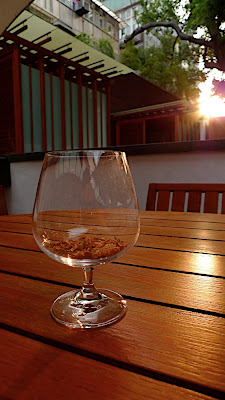## 2008年10月5日 星期日

### Oct.04, 小林麵館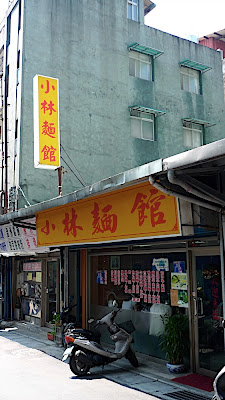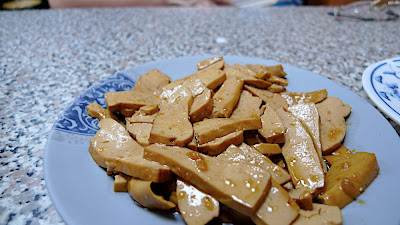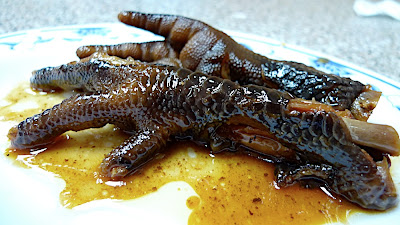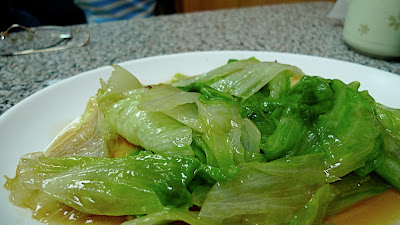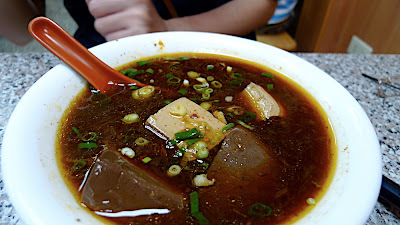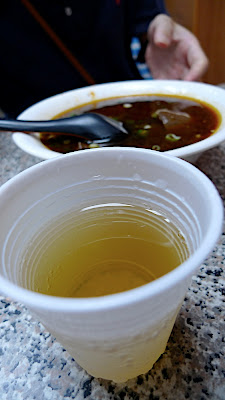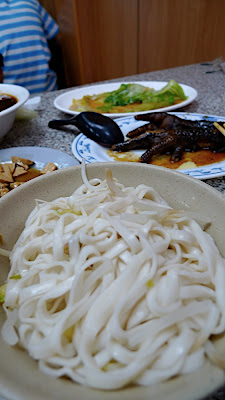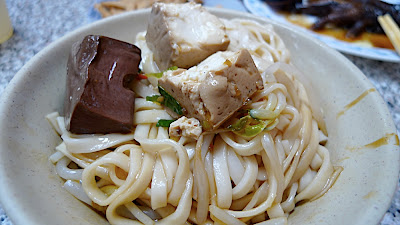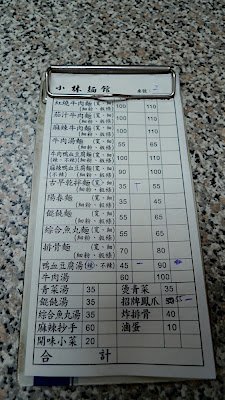## 2008年10月1日 星期三

### Sep.29, 小人物的感動，海角七號ps. 電影原聲帶快出吧…

EMBA雜誌編輯部／文